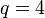# PSL(2,4) is isomorphic to A5

This article gives a proof/explanation of the equivalence of multiple definitions for the term alternating group:A5
View a complete list of pages giving proofs of equivalence of definitions

## Statement

Note that, due to the isomorphism between linear groups when degree power map is bijective, we know that over field:F4:$PGL(2,4) \cong PSL(2,4) \cong SL(2,4)$

where$PGL$ denotes the projective general linear group of degree two,$PSL$ denotes the projective special linear group of degree two, and$SL$ denotes the special linear group of degree two.

The claim is that all these isomorphic groups are isomorphic to alternating group:A5.

## Facts used

### Facts used for short proof using simplicity

The table below lists key facts used directly and explicitly in the proof. Fact numbers as used in the table may be referenced in the proof. This table need not list facts used indirectly, i.e., facts that are used to prove these facts, and it need not list facts used implicitly through assumptions embedded in the choice of terminology and language.
Fact no. Statement Qualitative description of how it is used
(S1) For$n \ge 2$, the group$A_n$ has order$n!/2$ Used to calculate the order of$A_5$ as$5!/2 = 60$
(S2) order formulas for linear groups of degree two. In particular,$SL(2,q)$ has order$q^3 - q$ The group$PSL(2,4) \cong SL(2,4) \cong PGL(2,4)$ has order$4^3 - 4 = 60$
(S3) A5 is the unique simple non-abelian group of smallest order:$A_5$ is a simple non-abelian group of smallest order and any simple non-abelian group of the same order is isomorphic to$A_5$. Used to force any other simple non-abelian group of that order to be isomorphic to it.
(S4) Projective special linear group is simple (non-abelian) with some exceptions for small sizes and degrees$PSL(2,4)$ is simple.

### Facts used for proof with explicit isomorphism

Fact no. Statement Qualitative description of how it is used
(E1) For$n \ge 2$, the group$S_n$ has order$n!$ Used to calculate the order of$S_5$ as$5! = 120$
(E2) Equivalence of definitions of size of projective space Used to calculate size of projective space of dimension one over field with four elements.
(E3) Order formulas for linear groups of degree two . In particular,$SL(2,q)$ has order$q^3 - q$ The group$PSL(2,4) \cong SL(2,4) \cong PGL(2,4)$ has order$4^3 - 4 = 60$
(E4) The only subgroup of order 60 in symmetric group:S5 is alternating group:A5 as A5 in S5. For more, see subgroup structure of symmetric group:S5. Determining the image of an injective group homomorphism to show isomorphism to the image.

## Proof

### Direct proof

The proof follows directly by combining (S1)-(S4) as follows: (S1) and (S2) establish that$A_5$ and$PSL(2,4)$ both have order 60. (S3) and (S4) establish that both are simple non-abelian, but$A_5$ is also the only one up to isomorphism for order 60 by (S3). This forces them to be isomorphic.

### Explicit bijection

Step no. Assertion/construction Facts used Previous steps used Explanation
1 For any field$k$, there is a natural faithful group action of$PGL(2,k)$ on$\mathbb{P}^1(k)$, the set of lines through the origin in$k^2$, and hence an injective group homomorphism from$PGL(2,k)$ to the symmetric group on$\mathbb{P}^1(k)$. Follows from definitions.
2 For$k$ the field of size$q$,$\mathbb{P}^1(k)$ has size$q + 1$ and thus the symmetric group on it has size$(q + 1)!$. (E1)
3 For$k$ the field of size$q = 4$,$PGL(2,k) =PGL(2,4)$ has order$4^3 - 3 = 60$ and$\operatorname{Sym}(\mathbb{P}^1(k))$ is the symmetric group of degree$4 + 1 = 5$ and has order$5! = 120$. (E2), (E3) Steps (2), (3) Plug in and evaluate.
4 The only subgroup of$\operatorname{Sym}(\mathbb{P}^1(k))$ of order 60 is alternating group:A5 as A5 in S5. (E4)
5 For$k$ the field of size$q = 4$, the homomorphism of Step (1) gives an injective group homomorphism from$PGL(2,4)$ to$S_5$ whose image is the subgroup$A_5$. In particular,$PGL(2,4)$ is isomorphic to$A_5$. Steps (1), (3), (4) By Steps (1) and (3), we get an injective homomorphism from$PGL(2,4)$ to$S_5$ whose image has order the same as$PGL(2,4)$, which is 60. By Step (4), the only possibility for the image is the subgroup$A_5$, completing the proof.